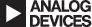#Clock Tool Prototype

$$\huge{\cssId{snrSym}{SNR} = {-20\log_{10}\sqrt{(2\pi \cssId{freqSym}{f_{a}}\cssId{tjrmsSym}{t_{j rms}})^2 + {2 \over 3}\; {({1 + \cssId{epsilonSym}{\epsilon} \over 2^\cssId{numBitsSym}{N}})^2} + ({2\sqrt{2}\cssId{VnoiseSym}{V_{NOISErms}} \over 2^\cssId{numBits2Sym}{N}})^2}} + \cssId{inputLevelSym}{level}}$$
$$\huge{\cssId{pfsSym}{P_{FS(dBm)}} = {({\cssId{VppSym}{V_{p-p}} \over 2\sqrt{2}}\;)^2 \over 2\cssId{resistanceSym}{R}}}$$
$$\huge{\cssId{noiseFigureSym}{NF} = \cssId{pfs2Sym}{P_{FS(dBm)}} + 174dBm - \cssId{snr2Sym}{SNR} - 10log_{10}{\cssId{bandWidthSym}{B}}}$$

## SNR Graph

### Input

*Peak-to-Peak Voltage (V p-p):

*Input 3dB Bandwidth (MHz):

*Resistance (Ohms):

Input Level (dBFS):

Clock Jitter (fs):

Average DNL (LSB):

Effective Input Noise (LSB):

Frequency Range (MHz):

SNR Range (dBFS):

Auto

Max Signal (MHz):

### Output

SNR at Max Signal:

Max Signal SNR Loss:

*Full Scale Power:

*Noise Figure at Max Signal:

*work in progress

## Calculate Specific

Calculate For

SNR (dBFS):

Input Level (dBFS):

Clock Jitter (fs):

Input Frequency (MHz):